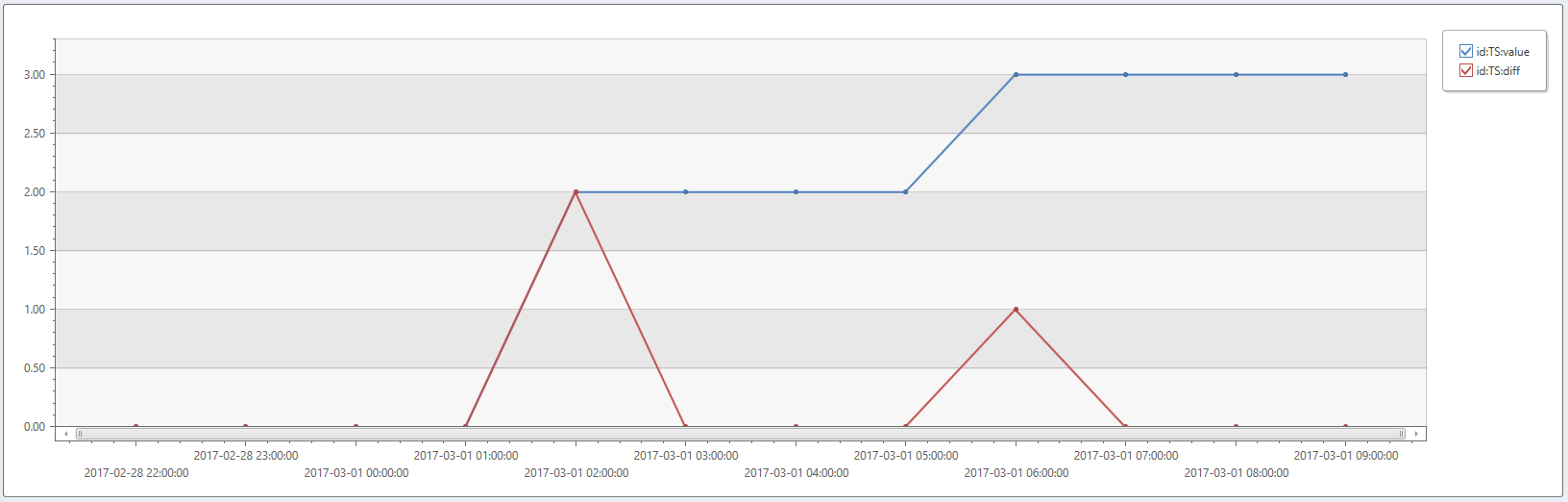# series_fir()

Applies a Finite Impulse Response (FIR) filter on a series.

The function takes an expression containing a dynamic numerical array as input and applies a Finite Impulse Response filter. By specifying the `filter` coefficients, it can be used for calculating a moving average, smoothing, change-detection, and many more use cases. The function takes the column containing the dynamic array and a static dynamic array of the filter's coefficients as input, and applies the filter on the column. It outputs a new dynamic array column, containing the filtered output.

## Syntax

`series_fir(`series`,` filter [`,` normalize[`,` center]]`)`

## Parameters

Name Type Required Description
series dynamic An array of numeric values.
filter synamic An array of numeric values containing the coefficients of the filter.
normalize bool Indicates whether the filter should be normalized. That is, divided by the sum of the coefficients. If filter contains negative values, then normalize must be specified as `false`, otherwise result will be `null`. If not specified, then a default value of `true` is assumed, depending on the presence of negative values in the filter. If filter contains at least one negative value, then normalize is assumed to be `false`.
center bool Indicates whether the filter is applied symmetrically on a time window before and after the current point, or on a time window from the current point backwards. By default, center is `false`, which fits the scenario of streaming data so that we can only apply the filter on the current and older points. However, for ad-hoc processing you can set it to `true`, keeping it synchronized with the time series. See examples below. This parameter controls the filter’s group delay.

Tip

Normalization is a convenient way to make sure that the sum of the coefficients is 1. When normalized is `true`, the filter doesn't amplify or attenuate the series. For example, the moving average of four bins could be specified by filter=[1,1,1,1] and normalized=`true`, which is simpler than typing [0.25,0.25.0.25,0.25].

## Returns

A new dynamic array column containing the filtered output.

## Examples

• Calculate a moving average of five points by setting filter=[1,1,1,1,1] and normalize=`true` (default). Note the effect of center=`false` (default) vs. `true`:
``````range t from bin(now(), 1h) - 23h to bin(now(), 1h) step 1h
| summarize t=make_list(t)
| project
id='TS',
val=dynamic([0, 0, 0, 0, 0, 0, 0, 0, 0, 10, 20, 40, 100, 40, 20, 10, 0, 0, 0, 0, 0, 0, 0, 0]),
t
| extend
5h_MovingAvg=series_fir(val, dynamic([1, 1, 1, 1, 1])),
5h_MovingAvg_centered=series_fir(val, dynamic([1, 1, 1, 1, 1]), true, true)
| render timechart
``````

This query returns:
5h_MovingAvg: Five points moving average filter. The spike is smoothed and its peak shifted by (5-1)/2 = 2h.
5h_MovingAvg_centered: Same, but by setting `center=true`, the peak stays in its original location.• To calculate the difference between a point and its preceding one, set filter=[1,-1].
``````range t from bin(now(), 1h) - 11h to bin(now(), 1h) step 1h
| summarize t=make_list(t)
| project id='TS', t, value=dynamic([0, 0, 0, 0, 2, 2, 2, 2, 3, 3, 3, 3])
| extend diff=series_fir(value, dynamic([1, -1]), false, false)
| render timechart
``````